FreeBuf.COM网络安全行业门户，每日发布专业的安全资讯、技术剖析。FreeBuf+小程序

2020-06-14 09:00:59

y-> 比如用户名称/id编号/权限简称

### 2.定位算法-动态调试

2.1在web站点上进行登陆,并抓包, 看到路径/member/index_do.php

2.2分析index_do.php (dedecms路由很简单, 路径直接对应到了文件),在登陆接口处下断点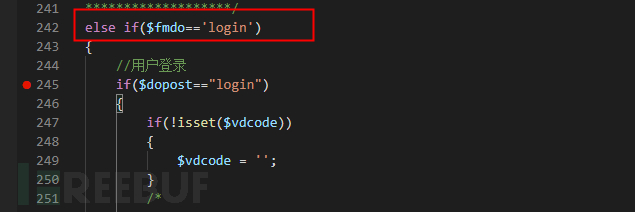2.3大致浏览整个函数, 并没有发现设置cookie的操作(php原型函数-setcookie),但是发现了检查账号的函数, 跟入进去: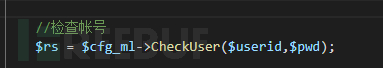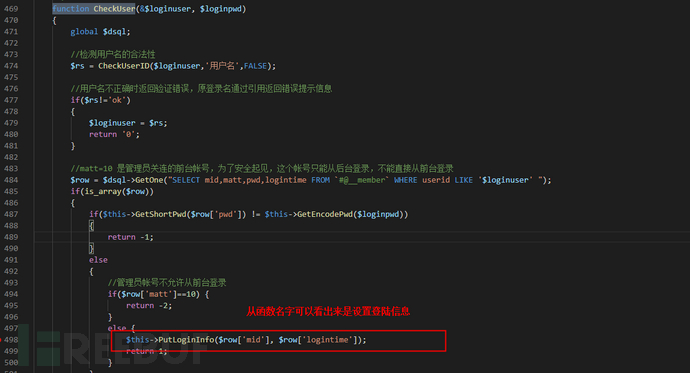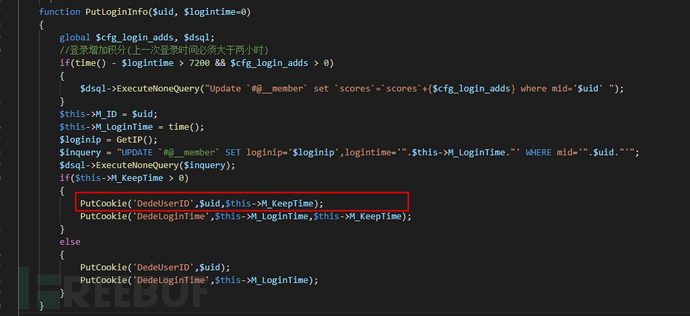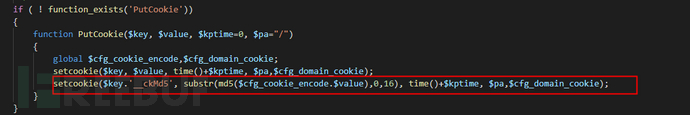2.4关键字段

``DedeUserID=7; DedeUserID_ckMd5=4d0db47b3ba3fef5;DedeUserID=用户ID; DedeUserIDckMd5 = substr(md5(\$cfgcookie_encode.用户ID),0,16)``

## 二、 Root Key生成算法

### 1. 代码定位

1.1 全文查找以下cfgcookieencode,发现在config.cache.inc.php存储有: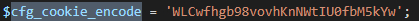这个值和我们在上面断点看到的值一样,大概率可以判断,应该属于一个固定值.

1.2 全局找一下有哪些地方操作了config.cache.inc.php,看是哪个函数写入了这个值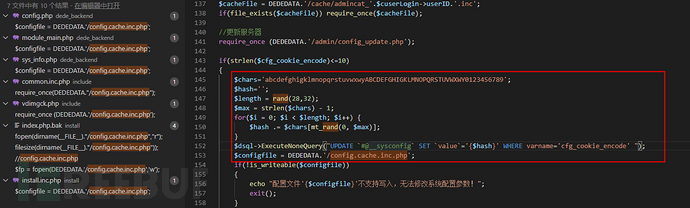1.3 继续定位到install.php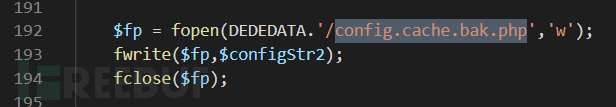在上下文找到了同样的root key生成算法: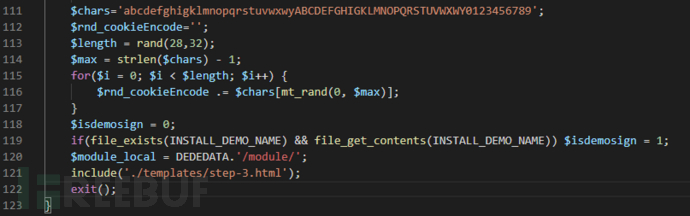在安装界面的时候其实会显示出来给我们: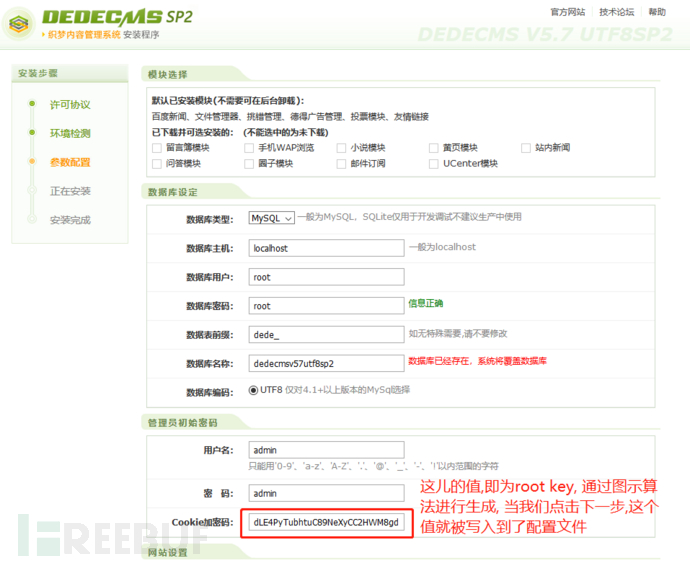1.4 根据1.2, 1.3可知Root Key算法如下:

``\$chars='abcdefghigklmnopqrstuvwxwyABCDEFGHIGKLMNOPQRSTUVWXWY0123456789';    \$max = strlen(\$chars) - 1;        \$length = rand(28,32);    \$root_key='';    for(\$i = 0; \$i < \$length; \$i++) {        \$root_key .= \$chars[mt_rand(0, \$max)];    }``

### 2. 强度分析

2.1 套用结论

4.1 影响随机数生成的因素为两个: 1. 种子 2. 次数

4.4 种子区间为0到0xffffffff

4.6 同一进程下,先后被调用的rand和mt_rand, 在未播种的前提下, 会使用同一个随机种子

62^28 + 62^29 + 62^30 + 62^31 + 62^32

2.2 遍历全部rootkey耗时

``<?php    \$start = microtime(true);    for (\$i = 0; \$i < 10000000; ++\$i) {        ;    }    \$total_1 = microtime(true) - \$start;        \$start = microtime(true);    for (\$i = 0; \$i < 10000000; ++\$i) {        md5("111111111111111111111111111111");    }    \$total_2 = microtime(true) - \$start;        \$start = microtime(true);    for (\$i = 0; \$i < 10000000; ++\$i) {        substr(md5("111111111111111111111111111111"),0,16);    }    \$total_3 = microtime(true) - \$start;        \$start = microtime(true);    \$chars='abcdefghigklmnopqrstuvwxwyABCDEFGHIGKLMNOPQRSTUVWXWY0123456789';    \$max = strlen(\$chars) - 1;    for (\$i = 0; \$i < 1000000; ++\$i) {        \$hash='';        \$length = rand(28,32);        for(\$y = 0; \$y < \$length; \$y++) {         \$hash .= \$chars[mt_rand(0, \$max)];        }    }    \$total_4 = microtime(true) - \$start;        echo (\$total_2 - \$total_1);    echo "\n";    echo (\$total_3 - \$total_1);    echo "\n";    echo (\$total_4 - \$total_1);    echo "\n";    ?>``

3.9920189380646 //10^7次md5() 用时

7.0076858997345 //10^7次substr(md5()) 用时

8.376072883606 // 10^6次生成key 用时

((8.376072883606/10^6) * (2^32)) / 3600 ≈ 10 hour

*本文作者：光通天下无患实验室  Djerryz，转载请注明来自FreeBuf.COM

# Cookie # rootkey # 随机算法

+ 收入我的专辑
+ 加入我的收藏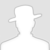\
• 0 文章数
• 0 评论数
• 0 关注者